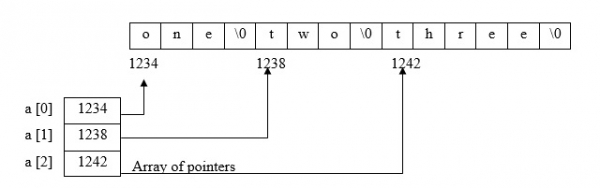# C program to print array of pointers to strings and their address

First, let us understand what are the arrays of pointers in C programming language.

## Arrays of pointers: (to strings)

• It is an array whose elements are ptrs to the base add of the string.

• It is declared and initialized as follows −

char *a[ ] = {"one", "two", "three"};

Here, a is a pointer to the base add of string "one".

a is a pointer to the base add of string "two".

a is a pointer to the base add of string "three".The advantages of array of pointers are explained below −

• Unlink the two dimensional array of characters, in array of strings and in array of pointers to strings, there is no fixed memory size for storage.

• The strings occupy only as many bytes as required hence, there is no wastage of space.

## Example

The C program demonstrating the concept of printing array of pointers to string and the addresses too is given below −

#include<stdio.h>
#include<string.h>
void main(){
//Declaring string and pointers, for loop variable//
int i;
char *a={"One","Two","Three","Four","Five"};
//Printing values within each string location using for loop//
printf("The values in every string location are : ");
for(i=0;i<5;i++){
printf("%s",a[i]);
}
//Printing addresses within each string location using for loop//
printf("The address locations of every string values are : ");
for(i=0;i<5;i++){
printf("%d",a[i]);
}
}

## Output

When the above program is executed, it produces the following result −

The values in every string location are:
One
Two
Three
Four
Five
The address locations of every string values are:
4210688
4210692
4210696
4210702
4210707

## Example 2

Let’s consider another example.

Stated below is a C program demonstrating the concept of array of pointers to string −

#include<stdio.h>
#include<string.h>
void main(){
//Declaring string and pointers//
char string="TutorialPoint";
char *pointer = string;
//Printing the string using pointer//
printf("The string is : ");
while(*pointer!='\0'){
printf("%s",*pointer);
pointer++;
}
}

## Output

When the above program is executed, it produces the following result −

The string is: TutorialPoint